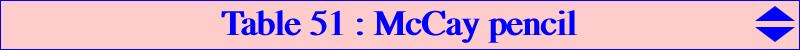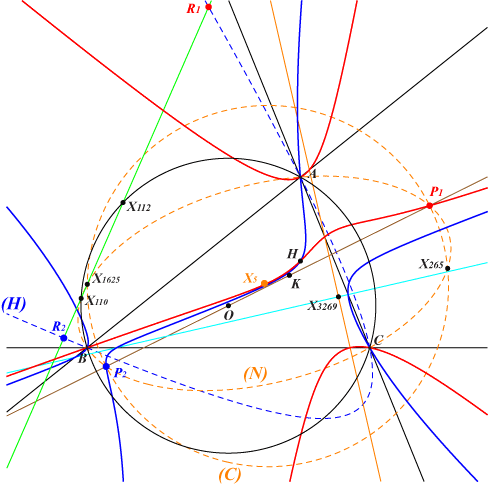The McCay pencil is generated by the McCay cubic K003 and the McCay orthic cubic K049. These two cubics are stelloidal pKs with same infinite points and radial center X(2), X(51) respectively. The pencil contains the degenerate cubic which is the union of the line at infinity and the Kiepert hyperbola, this being an isogonal nK0 with root X(2). This latter cubic and K003 are therefore two other cubics which generate the pencil. It follows that the remaining base points of the pencil are X(4) and the imaginary foci of the Brocard ellipse, the common points of the Brocard axis and the Kiepert hyperbola. Hence each cubic K(P) of the pencil is determined by its third real intersection P with the Brocard axis. Obviously, K(X3) = K003, K(X52) = K049 and K(X511) is the decomposed cubic. The pencil contains no other pK and two other quite complicated nK0s denoted (K1), (K2) described below. Now, if Q is the midpoint of X(3)-P then K(P) is the cubic spK(X3, Q) of CL055 with radial center X on the line X(2), X(51). The abscissa of X in (X2, X51) is that of Q in (X3, X389). This gives two triads of collinear points namely X(4), Q, X and X(5), P, X. Recall that P, Q both lie on the Brocard axis. It follows that X is the centroid of triangle X(3)X(4)P. Note that the Hessian H(P) of K(P) is a focal cubic with singular focus X. See K048, the Hessian of K003. The following table shows a selection of the most remarkable cubics K(P). See CL006 for other stelloids.K(P) Q X P remarks - X(511) X(511) X(511) decomposed cubic K003 X(3) X(2) X(3) K049 X(389) X(51) X(52) K115 X(52) X(3060) X(6243) K268 X(13348) X(3819) X(15644) K516 X(39) X(262) X(3095) also contains the Brocard points K643 X(182) X(14561) X(6) X on X(5)X(6) K708 X(6) X(14853) X(1351) X on X(4)X(6) K1139 X(9730) X(5640) X(568) X on X(6)X(110)The two cubics (K1), (K2)The circumconic (N) – sometimes called Johnson circumconic – with center X(5) passing through X(110), X(265), X(1625) meets the Brocard axis at P1, P2 which are the two points P as above corresponding to the two nK0s of the pencil. Note that these points P1, P2 also lie on the circle (C) with center on the Brocard axis and passing through X(265), X(1625). The roots R1, R2 are the common points of the line X(110)X(112) and the circum-hyperbola (H) with perspector X(3269) passing through X(520), X(523), X(647), X(1304) which is the X(184)-isoconjugate of the line. The trilinear polars of R1, R2 are perpendicular at X(3269). They are parallel to the asymptotes of the rectangular circum-hyperbola passing through X(51), X(184), etc. The poles Ω1, Ω2 of the two cubics are the intersections of the trilinear polar of X(112) and the circum-conic with perspector X(51). Recall that the radial centers X1, X2 lie on the line X(2)X(51) and are the centroids of OHP1, OHP2 respectively.All these points are unlisted in ETC with SEARCH numbers : P1, P2 : -1.378378336750758 and 0.2940277831621287 Ω1, Ω2 : 0.257195393615944 and -0.102766799323409 R1, R2 : -2.10308251616673 and 6.93744576712799 X1, X2 : -0.0908782844936497 and 0.466590422143979